# Dynamic metabolic modelling of nitrifying consortia¶

#### Christopher E. Lawson1 ([email protected]), Lise O. Aagesen2 ([email protected]), and Eric C. McCurry3 ([email protected])¶

1 Civil and Environmental Engineering, University of Wisconsin-Madison, 2 Biomedical Engineering, Technical University of Denmark, 3 Chemical and Biological Engineering, University of Wisconsin-Madison

## 1. Introduction¶

A major goal of systems biology is to develop mathematical models that can simulate an organism's biochemical characteristics, or phenotype, based on its biological components (e.g. genes, enzymes, metabolites). A common approach taken by systems biologists to model the phenotype of microorganisms rely on constraint-based reconstruction and analysis (COBRA) methods that simulate the flow of metabolites through an organisms metabolic network to predict system properties, such as rates of biomass growth or chemical production (O'Brien et al., 2015). COBRA methods have been under development since the mid-1990s and have become powerful tools for enabling predictive biology. This project focuses on the application of COBRA methods, specifically, flux balance analysis (FBA) (Orth et al., 2010), to model the biochemical process of nitrification mediated by two different groups of microorganisms.

### 1.A. Microbiology and chemistry of nitrification¶

Nitrification is an important step in the global nitrogen cycle, where ammonia (NH3) is first oxidized to nitrite (NO2-) by ammonia oxidizing bacteria (AOB), and subsequently to nitrate (NO3-) by nitrite oxidizing bacteria (NOB). The process was discovered by Sergei Winogradsky in 1890 (Dworkin, 2012) and plays an important role in controlling the availability of nitrogen in soil and marine ecosystems, and removing ammonia from contaminated waters during water and wastewater treatment (see Nitrification primer).

In aerobic environments, the first step of nitrification (ammonia oxidation) it often done by bacteria affiliated with the genus Nitrosomonas, whereas the second step (nitrite oxidation) is often done by bacteria affiliated with the genus Nitrobacter. The overall chemical transformations of nitrification are shown below:

Step 1. $\hspace{1cm} 2 \hspace{0.1 cm} NH_3 + 3 \hspace{0.1 cm} O_2 \rightarrow 2 \hspace{0.1 cm} NO_2^- + 2 \hspace{0.1 cm} H_2O + 4 \hspace{0.1 cm} H^+ \hspace{1cm}$(Nitrosomonas)

Step 2. $\hspace{1cm} 2 \hspace{0.1 cm} NO_2^- + O_2 \rightarrow 2 \hspace{0.1 cm} NO_3^- \hspace{4.4cm}$(Nitrobacter)

### 1.B. Problem statement¶

The ultimate goal of this project was to predict the population dynamics and nutrient cycling (NH3, NO2-, and NO3-) of a Nitrosomonas/Nitrobacter co-culture performing nitrification in a batch bioreactor, based on each organisms metabolic network stoichiometry and nutrient uptake kinetics (Figure 1). The ability to predict and simulate nitrification is essential for the design of biological wastewater treatment systems, and is a classic problem in environmental engineering. Moreover, being able to predict the nitrification process is critical to managing the global nitrogen cycle, which has been identified as a grand challenge for the 21st century by the National Academy of Engineering (NAE).

**Figure 1** (from [Louca and Doebeli, 2015](http://www.ncbi.nlm.nih.gov/pubmed/26404023))Model development was conducted in a stepwise manner to achieve our ultimate project goal. The growth of each organism was first modelled at steady-state to determine their maximum growth rate and nutrient uptake/export rates for NH3, NO2-, and NO3-. A sensitivity and robustness analysis was also conducted to evaluate how each organisms growth was impacted by intracellular metabolite imbalances and changes in nutrient uptake rates. Subsequently, resulting steady-state models were solved over multiple timepoints to simulate the population dynamics and chemical transformations occuring in a bioreactor cycle.

Because several species are known to carry out the nitrification process, we were also interested in predicting the outcome of competiton experiments between different species of Nitrobacter involved in the second step of nitrification (NO2- oxidation). This was simulated using the dynamic microbial community model, where each species started at the same initial concentration and their abundance at the end of the cycle was determined.

The network stoichiometry used for Nitrosomonas and Nitrobacter were adapted from (Louca and Doebeli, 2015); kinetic parameters for nutrient uptake and affinity were adapted from Laanbroek et al., 1994 and Nowka et al., 2015.

## 2. Mathematical Model and Solutions¶

### 2.A. Metabolic networks: stoichiometry & kinetics¶

Metabolic networks are comprised of compounds (nodes) and biochemical reactions (edges). An organisms metabolic network determines its physiological and biochemical properties, including what nutrients it consumes, how it generates energy (e.g. ATP) and reducing power (electrons in the form of NADH), and how it synthesizes biomass (i.e. proteins, lipids, nucleic acids, carbohydrates). The proportion of reactants to products for a given biochemical reaction is termed stoichiometry. Metabolic networks can be represented mathematically as a matrix of compounds (rows) and reactions (columns), where the matrix entries represent stoichiometric coefficients. Figure 2 provides a conceptual example of how a metabolic network is converted to a mathematical representation.

Figure 2 (from O'Brien et al., 2015)Nitrifier metabolism and stoichiometry
Nitrifying bacteria, such as Nitrosomonas and Nitrobacter, are "chemolithoautotrophic" microorganisms, meaning they grow using CO2 as a carbon source (similar to plants) and use inorganic substrates (of mineral origin) to generate energy and reducing power (electrons) for biosynthesis and cellular maintenance. For nitrifying bacteria, inorganic nitrogen compounds are used as primary substrates: Nitrosomonas use NH3, whereas Nitrobacter use NO2-. Like humans, nitrifying bacteria also require O2 as an electron acceptor for energy production via aerobic respiration.

For this project, simplifed metabolic networks for Nitrosomonas and Nitrobacter were used to model cell growth (Louca and Doebeli, 2015). Networks were first converted to stoichiometric tables in excel and saved as csv files. Resulting tables were then read into Julia as matrices and stored as NamedArrays. The total number of compounds and reactions for each metabolic network are provided on Table 1.

Table 1

Model Reactions Compounds
Nitrosomonas 30 28
Nitrobacter 26 24

Figure 3 shows the metabolic network for Nitrosomonas used in this project. The details of the metabolic network for Nitrobacter can be found in the supplementary information.

Figure 3 (from Perez-Garcia et al., 2014)Monod kinetics
The kinetics of substrate uptake for Nitrosomonas and Nitrobacter were modelled using the Monod equation:

$\hspace{1.7cm} r^k_{uptake,i} = V_{m,i}^k(\frac{C^k_i}{K_{m,i}^k + C^k_i}) \hspace{0.5cm} (1)$

Where,

$r^k_{uptake,i}$ = Uptake rate of substrate i by organism k
$V_{m,i}^k$ = maximum uptake rate of substrate i for organism k
$K_{m,i}^k$ = half-saturation coefficient of substrate i for organism k
$C^k_i$ = Extracellular concentration of substrate i available to organism k

The Monod equation is commonly used to model kinetic limitations on substrate uptake by microorganisms. Under non-limiting conditions, substrate uptake occurs at the maximum rate, Vm. Under substrate-limiting conditons, the substrate uptake rate is based on the organisms affinity for the substrate (Km) and the substrate concentration (Ci).

For our modelling purposes, kinetic constraints were only imposed on the primary substrates for each organism; NH3 for Nitrosomonas and NO2- for Nitrobacter. All other nutrients required for growth (e.g. phosphorous, CO2, O2, etc.) were assumed to be non-limiting (see Section 2.B. for further details).

In :
#Nitrosomonas stoichiometry and kinetics
using NamedArrays

#read stoichiometry table as matrix
rawNS = readdlm("Lawson_Nitrosomonas.csv",',');
(ms,ns) = size(rawNS)

#range of reactions and metabolites in matrix
ns_reactions = 2:ns
ns_metabolites = 2:ms

#names of reactions and metabolites
reactionsNS = rawNS[1,ns_reactions][:]
metabolitesNS = rawNS[ns_metabolites,1][:]

#collect exchange reactions
EXrxnsNS = []
for i in reactionsNS
if ismatch(r"EX_",i) == true
push!(EXrxnsNS,i)
end
end

#collect intracellular reactions
INTrxnNS = []
for i in reactionsNS
if ismatch(r"EX_|T_",i) == false
push!(INTrxnNS,i)
end
end

#stochiometry matrix
stoicmatrixNS = NamedArray( Array{Float64}(rawNS[ns_metabolites,ns_reactions]), (metabolitesNS,reactionsNS),("metabolitesNS","reactionsNS") )
println(stoicmatrixNS)

#Nitrosomonas europaea kinetics
KdNS = 0.04       #Biomass decay coefficient (days-1)
VmNS = 2.14e-13  #Nitrosomonas max growth rate (mol NH3/cell-d)
KmNS = 1.050     #Half-saturation value for NH3 (mM)
wt_NS = 3e-13    #cell weight (g DW/cell)
Vm_NS = (VmNS*1000)/wt_NS  #(mmol/gDW-d)
MW = 1198/1000   #gDW/mmol, calculated from Smatrix ;

28x30 NamedArray{Float64,2}:
metabolitesNS \ reactionsNS R_biomassNS R_maintNS   …  EX_CO2      EX_H2O
ADP_c                       15.0        1.0         …  0.0         0.0
ATP_c                       -15.0       -1.0        …  0.0         0.0
CO2_c                       -5.0        0.0         …  -1.0        0.0
H_c                         -7.0        1.0         …  0.0         0.0
H_p                         0.0         0.0         …  0.0         0.0
H2O_c                       0.0         -1.0        …  0.0         -1.0
HNO2_NO2_c                  0.0         0.0         …  0.0         0.0
HNO2_NO2_e                  0.0         0.0         …  0.0         0.0
N2O_c                       0.0         0.0         …  0.0         0.0
NAD_c                       10.0        0.0         …  0.0         0.0
NADH_c                      -12.0       0.0         …  0.0         0.0
NH2OH_c                     0.0         0.0         …  0.0         0.0
⋮           ⋮           ⋱  ⋮           ⋮
O2_c                        4.0         0.0         …  0.0         0.0
Pi_c                        15.0        1.0         …  0.0         0.0
protein_c                   -0.25       0.0         …  0.0         0.0
maint_c                     -32.0       1.0         …  0.0         0.0
Q8H2_c                      0.0         0.0         …  0.0         0.0
Q8_c                        0.0         0.0         …  0.0         0.0
Cyt554e_c                   0.0         0.0         …  0.0         0.0
Cyt554_c                    0.0         0.0         …  0.0         0.0
Cyt552me_c                  0.0         0.0         …  0.0         0.0
Cyt552m_c                   0.0         0.0         …  0.0         0.0
Cyt552e_c                   0.0         0.0         …  0.0         0.0
Cyt552_c                    0.0         0.0         …  0.0         0.0


In :
#Nitrobacter stoichiometry and kinetics
using NamedArrays

#read stoichiometry table as matrix
rawNB = readdlm("Lawson_Nitrobacter.csv",',')
(mb,nb) = size(rawNB)

#range of reactions and metabolites in matrix
nb_reactions = 2:nb
nb_metabolites = 2:mb

#names of reactions and metabolites
reactionsNB = rawNB[1,nb_reactions][:]
metabolitesNB = rawNB[nb_metabolites,1][:]

#collect exchange reactions
EXrxnsNB = []
for i in reactionsNB
if ismatch(r"EX_",i) == true
push!(EXrxnsNB,i)
end
end

#collect intracellular reactions
INTrxnNB = []
for i in reactionsNB
if ismatch(r"EX_|T_",i) == false
push!(INTrxnNB,i)
end
end

#stochiometry matrix
stoicmatrixNB = NamedArray( Array{Float64}(rawNB[nb_metabolites,nb_reactions]), (metabolitesNB,reactionsNB),("metabolitesNB","reactionsNB"))
println(stoicmatrixNB)

#Nitrobacter winogradskyi kinetics
KdNB = 0.04      #Biomass decay coefficient (days-1)
VmNB = 8.88e-14  #Nitrobacter max growth rate (mol NO2/cell-d)
KmNB = 0.309     #Half-saturation value for NO2 (mM)
wt_NB = 4e-13    #cell weight (g DW/cell)
Vm_NB = (VmNB*1000)/wt_NB #(mmol/gDW-d)
MW = 1198/1000   #gDW/mmol, calculated from Smatrix;

24x26 NamedArray{Float64,2}:
metabolitesNB \ reactionsNB  …
ADP_c                        …
ATP_c                        …
CO2_c                        …
Cytc_550ox_c                 …
Cytc_550red_c                …
H_c                          …
H_p                          …
H2O_c                        …
HNO2_NO2_c                   …
HNO2_NO2_e                   …
HNO3_NO3_c                   …
HNO3_NO3_e                   …
NAD_c                        …
NADH_c                       …
NH3_NH4_c                    …
NH3_NH4_e                    …
NO_c                         …
O2_c                         …
Pi_c                         …
protein_c                    …
UQ_c                         …
UQH2_c                       …
maint_c                      …
biomass                      …



### 2.B. Flux balance analysis¶

Flux balance analysis (FBA) is a mathematical optimization approach for analyzing the flow of metabolites through a metabolic network (Orth et al., 2010). Here, flux is defined as the molar flow rate of molecules through a given reaction per unit biomass [mmol /g of dry cell weight(DW) /hour]. Both Nitrosomonas' and Nitrobacter's metabolic network were initially analyzed using FBA to determine their maximum growth rate and corresponding uptake/export rates for NH3, NO2-, and NO3- at steady-state. The decision variables for the FBA problem were the metabolic fluxes through each reaction. The objective function was to maximize flux through the biomass synthesis reaction (i.e. maximize cell growth), subject to the following constraints:

• Mass conservation: the stoichiometry matrix imposes flux balance constraints on the network, such that the total production rate of a given metabolite must equal its total consumption rate (i.e. steady-state constraint).
• Upper and lower flux bounds on each reaction.
• Substrate uptake rate constraints set by experimentally determined kinetic parameters.

Figure 4 provides a conceptual image of the biomass objective function.

Figure 4 (from O'Brien et al., 2015)The problem is typically written as:

\begin{aligned} \underset{v \in \mathbb{R^n} }{\text{maximize}}\qquad& \mu^k = c^Tv_j^k = v^k_{biomass} \hspace{0.5cm} (2)\\ \text{subject to:}\qquad& \sum S^k_{i,j}v^k_j = b_i && \forall \hspace{0.1cm} i \in I \hspace{0.5cm} (3)\\ & LB^k_j \le v^k_j \le UB^k_j && \forall \hspace{0.1cm} j \in J \hspace{0.5cm} (4)\\ & v^k_{uptake,i} \le V_{m,i}^k(\frac{C^k_i}{K_{m,i}^k + C^k_i}) && \hspace{0.1cm} i = NH_3, NO_2^- \hspace{0.5cm} (5)\\ \end{aligned}

Where,

Sets
$K$ = Set of organisms
$J^k$ = Set of reactions in metabolic network of organism k
$I^k$ = Set of metabolites in metabolic network of organism k

Parameters
$S^k_{i,j}$ = Stoichiometric coefficients of metabolite $i \in I^k$ in the reaction $j \in J^k$
$LB^k_j$ and $UB^k_j$ = Lower and upper flux bound on reaction $j \in J^k$ for community member k
$V_{m,i}^k$ = maximum uptake rate of substrate i for organism k
$K_{m,i}^k$ = half-saturation coefficient of substrate i for organism k
$C^k_i$ = Extracellular concentration of substrate i available to organism k
$c^T$ = cost array containing all zeros except for a 1 in the position corresponding to the biomass flux reaction
$b_i$ = the rate of accumulation/depletion of metabolite i, assumed here to be zero (i.e. steady-state)

Variables
$v^k_j$ = Metabolic flux through reaction j in community member k
$v^k_{uptake,i}$ = substrate uptake flux by community member k

Because the righthand side of Eq. 5 reduces to a constant, the problem stated above can be solved using linear programming. A function for performing FBA is coded in Julia + JuMP below.

Dual problem and shadow prices
The sensitivity of FBA to flux imbalances can be captured by the dual problem and its corresponding variables (shadow prices):

\begin{aligned} \underset{\lambda \in \mathbb{R^n},q_1\le0,q_2\ge0,\gamma\ge0 }{\text{minimize}}\qquad& \lambda^Tb_i + q_1^TLB + q_2^TUB + \gamma^Tr_i^k \hspace{0.5cm} (6)\\ \text{subject to:}\qquad& \sum \lambda^T_iS^k_{i,j} + q_{1,j}^T + q_{2,j}^T + \gamma^T_j= c^T && \forall \hspace{0.1cm} i \in I \hspace{0.5cm} (7)\\ \end{aligned}

Where,

$\lambda_i$ = the shadow prices for the steady-state constraints on metabolite i
$q_{1,j}$ = the shadow prices for the lower bound flux constraints on reaction j
$q_{2,j}$ = the shadow prices for the upper bound flux constraints on reaction j
$\gamma_j$ = the shadow prices for the substrate uptake constaints on reaction j
$r_i^k$ = the constant obtained from evaluating the right hand side of Eq. 5

The shadow prices for the metabolites ($\lambda_i$) are most important to us and quantify the "value" of each metabolite to the objective function; that is, how sensitive the objective function is to flux imbalances. A negative $\lambda_i$ means that adding metabolite i would increase the biomass growth rate. A positive $\lambda_i$ means that removing metabolite i would increase the biomass growth rate. A $\lambda_i$ of zero means that adding or removing metabolite i would have no affect on the biomass growth rate.

The dual values are automatically output from the FBA function coded below and are used in subsequent analysis detailed in this report.

Model assumptions
Our FBA model is based on two key assumptions:

1. Metabolic networks operate under steady-state conditions. That is, there is no intracellular accumulation or depletion of metabolites in the network. This assumption is justified becuase the rate of turnover of most metabolic intermediates is high relative to intracellular concentrations. Transient effects of metabolism due to perturbations are thus resolved quickly relative to the rate of cell growth.

2. The objective of a cell is to maximize its growth rate. While the use of appropriate objective functions (e.g. minimize ATP usage, minimize ribosome dilution, etc.) is currently an open topic in metabolic engineering, the maximization of growth assumption works well for microbial systems in suspended cell culture. Due to the exponential nature of cell growth, the fastest growing cells will always be dominate in the total cell population. In other words, rapidly growing phenotypes will always outcompete slower variants of the same species.

Other assumptions relating to the physical environment were also made. These include ignoring the effects of temperature, pH, and diffusion limitations on the chemical reactions considered in the metabolic network.

In :
using JuMP, Gurobi

#solve steady-state FBA model
function fbaNS(u,C)                 #set uptake rate (value) for reaction (key)
λNS = Dict()                    #dictionary of metabolite shadow prices
z = Model(solver=GurobiSolver(OutputFlag=0))
@variable(z, flux[reactionsNS]) #flux variables for each reaction
@variable(z, t[key in keys(u)]) #t equals absolute value of flux["r"]

#steady-state metabolite constraints
@constraint(z, stoichiometry[i in metabolitesNS], sum{flux[j]*stoicmatrixNS[i,j], j in reactionsNS} == 0)
@constraint(z, direction[i in INTrxnNS], flux[i] >= 0)    #Directionality constraints on reaction fluxes
@constraint(z, flux["EX_NO"] == 0)
@constraint(z, c1[key in keys(u)], t[key] <= u[key])      #uptake rate limited by nutrient availability
@constraint(z, c2[key in keys(u)], t[key] >= flux[key] )  #key sets exchange flux reaction
@constraint(z, c3[key in keys(u)], t[key] >= -flux[key] ) #key sets exchange flux reaction

#If nutrient concentration is 0, uptake flux must be zero
if C[:NH3] <= 0
@constraint(z, flux["EX_NH3"] >= 0 )
end
if C[:NO2] <= 0
@constraint(z, flux["EX_NO2"] >= 0 )
end
if C[:NO3] <= 0
@constraint(z, flux["EX_NO3"] >= 0 )
end

@objective(z, Max, flux["R_biomassNS"]) #maximize biomass growth
solve(z)

#output model solutions
global μ = (getobjectivevalue(z))
global rNH3 = getvalue(flux["EX_NH3"])
global rNO2 = getvalue(flux["EX_NO2"])
global rNO3 = getvalue(flux["EX_NO3"])
global fluxes = getvalue(flux)
for i in metabolitesNS
λNS[i] = getdual(stoichiometry[i])
end
global λNS

return μ, rNH3, rNO2, rNO3, fluxes, λNS
end

#Determine max growth rate
C1=Dict(:NH3=>100 ,:NO2=>0.0 ,:NO3=>0.0 )
rate = Vm_NS
u = Dict("EX_NH3" => rate)
outputNS = Dict(:a => [])
push!(outputNS[:a],fbaNS(u,C1)) #run model

#print model results
println("μ: ",μ, ", rNH3: ", rNH3,", rNO2: ",rNO2, ", rNO3: ",rNO3)

for i in λNS
#println(i) #shadow price for metabolite i
end

println("reaction fluxes: ")
for j in reactionsNS
println("$j: ",fluxes[j]) #fluxes end  μ: 10.853302903512107, rNH3: -713.3333333333334, rNO2: 702.4800304298212, rNO3: 0.0 reaction fluxes: R_biomassNS: 10.853302903512107 R_maintNS: 347.3056929123875 R_proteinNB: 2.713325725878027 R_ATP_synt: 534.2538354253837 R_amo: 702.4800304298212 R_HAO_noh: 702.4800304298212 R_HAO_no: 702.4800304298212 R_HAO_hno2: 702.4800304298212 R_Cyt554: 1404.9600608596425 R_Q8H2_synt: 1404.9600608596425 R_NADH_synt: 130.23963484214528 R_Cytbc1: 572.240395587676 R_Cytaa3: 572.240395587676 R_CytP460: 0.0 R_nir: 0.0 R_nor: 0.0 T_NH3: -713.3333333333334 T_NO2: 702.4800304298212 EX_O2: -945.1870166096107 EX_NH3: -713.3333333333334 EX_NO2: 702.4800304298212 EX_NO3: 0.0 EX_H: 425.72080639026217 EX_NO: 0.0 EX_N2O: 0.0 EX_Pi: 0.0 EX_ADP: 0.0 EX_NAD: -21.706605807024204 EX_CO2: -54.26651451756054 EX_H2O: 759.1885381006722  In : using JuMP, Gurobi #solve steady-state FBA model function fbaNB(u, C) #set uptake rate (u) for reaction (r) λNB = Dict() #dictionary of metabolite shadow prices z = Model(solver=GurobiSolver(OutputFlag=0)) @variable(z, flux[reactionsNB]) #flux variables (mmol/mgDW/day) @variable(z, t[key in keys(u)]) #t equals absolute value of flux["r"] #steady-state metabolite constraints @constraint(z, stoichiometry[i in metabolitesNB], sum{flux[j]*stoicmatrixNB[i,j], j in reactionsNB} == 0) @constraint(z, direction[i in INTrxnNB], flux[i] >= 0) #Directionality constraints on reaction fluxes @constraint(z, flux["EX_NO"] == 0) @constraint(z, c1[key in keys(u)], t[key] <= u[key]) #uptake rate limited by nutrient availability @constraint(z, c2[key in keys(u)], t[key] >= flux[key] ) #n equals exchange flux reaction @constraint(z, c3[key in keys(u)], t[key] >= -flux[key] ) #n equals exchange flux reaction #If nutrient concentration is 0, uptake flux must be zero if C[:NH3] <= 0 @constraint(z, flux["EX_NH3"] >= 0 ) end if C[:NO2] <= 0 @constraint(z, flux["EX_NO2"] >= 0 ) end if C[:NO3] <= 0 @constraint(z, flux["EX_NO3"] >= 0 ) end @objective(z, Max, flux["R_biomassNB"]) #maximize biomass growth solve(z) #output model solutions global μ = (getobjectivevalue(z)) global rNH3 = getvalue(flux["EX_NH3"]) global rNO2 = getvalue(flux["EX_NO2"]) global rNO3 = getvalue(flux["EX_NO3"]) global fluxes = getvalue(flux) for i in metabolitesNB λNB[i] = getdual(stoichiometry[i]) end global λNB return μ, rNH3, rNO2, rNO3, fluxes , λNB end #Determine max growth rate C2=Dict(:NH3=>0.0,:NO2=>1.0,:NO3=>0.0) u = Dict("EX_NO2" => Vm_NB) test = Dict(:b => []) push!(test[:b],fbaNB(u, C2)) #run model #print results println("μ: ",μ, ", rNH3: ", rNH3,", rNO2: ",rNO2, ", rNO3: ",rNO3) for i in λNB #println(i) #metabolite shadow prices end println("reaction fluxes:") for j in reactionsNB println("$j: ",fluxes[j]) #fluxes
end

μ: 0.6737016345954892, rNH3: 0.0, rNO2: -221.99999999999997, rNO3: 221.32629836540448
reaction fluxes:
R_biomassNB: 0.6737016345954892
R_maintNB: 21.558452307055653
R_proteinNB: 0.1684254086488723
Jnrj8: 221.32629836540448
Jnrj9: 20.211049037864672
Jnrj10: 10.105524518932336
Jnrj11: 0.0
Jtermox_dissip_Nitrobacter: 211.22077384647216
JNAD: 10.105524518932336
JATP: 33.16296296296296
R_nirBD: 0.6737016345954892
T_NH3: 0.0
T_NO2: -221.99999999999997
T_NO3: 221.32629836540448
EX_O2: -102.91558038485412
EX_NH3: 0.0
EX_NO2: -221.99999999999997
EX_NO3: 221.32629836540448
EX_H: 25.752244982412606
EX_NO: 0.0
EX_Pi: 0.0
EX_ADP: 0.0
EX_NAD: -1.347403269190977
EX_CO2: -3.368508172977446
EX_H2O: 2.8463894061659687
EX_biomass: 0.6737016345954892


### 2.C. Phenotype phase planes¶

Phenotypic phase plane (PhPP) analysis was conducted to determine the sensitivity of the objective function (biomass growth rate) to simultaneous changes in oxygen and ammonia (two key metabolites for growth). In PhPP analysis, two uptake fluxes are varied simultaneously on an x,y-plane and used to describe changes in the objective function (Edwards et al., 2002). The optimal biomass flux solutions are calculated for all points examined on this plane and the resulting regions or "phases" represent different metabolic network utilizations (i.e. phenotypes).

The PhPP can be defined using the shadow prices from the solutions to the dual FBA problems. Once all points have been evaluted in the two-dimensional plane, the shadow prices for all metabolites are calculated within this region and lines are drawn to demarcate regions of constant shadow prices. Figure 5 shows a conceptual PhPP.

Figure 5 (from Bernhard Ø. Palsson, UCSD,FBA lecture slides)The isoclines or contours of the phase plane represent the combination of uptake fluxes that result in the same objective value. The slope of the isocline can be calculated from the ratio of shadow prices for the metabolites corresponding to each uptake flux as follows:

$\hspace{1.7cm} \alpha = -\frac{\lambda_A}{\lambda_B} = -\frac{\frac{dZ}{db_A}}{\frac{dZ}{db_B}} = \frac{db_B}{db_A} \hspace{0.5cm} (8)$

Where,

$\alpha$ = the relative change in the objective function for the two examined exchange fluxes,
$\lambda$ = the shadow price of metabolite A or B, and
$\frac{dZ}{db}$ = the change or sensitivity of the objective function (Z) to changes in the availability of metabolites A or B.

Solution
PhPP analysis was only conducted for Nitrosomonas. Here, the maximal growth rate of Nitrosomonas was examined as a function of simultaneously varying the oxygen uptake and ammonia uptake fluxes. A line representing the optimal relationship between the two metabolic fluxes was also determined. This "line of optimality" (LO) was calculated by fixing the ammonia uptake flux along the x-axis and determining the optimal biomass flux using FBA. The code for PhPP is provided below in Julia + JuMP.

In :
#Phenotype Phase Plane Analysis (PhPP)

#inputs
nPts = 50     #number of points to plot in each dimension
range1 = 700  #range of reaction 1 to plot
range2 = 700  #range of reaction 2 to plot
rxnID = ["EX_NH3", "EX_O2"]
metID = ["NH3_NH4_e", "O2_c"]

#create empty vector for PhPP results
C3=Dict(:NH3=>100 ,:NO2=>100 ,:NO3=>100 )
b1 = collect(linspace(0, range1, nPts))
b2 = collect(linspace(0, range2, nPts))
b = Dict(rxnID => b1, rxnID => b2 )
PhPP = []
growthRates = []
shadowPrice1 = []
shadowPrice2 = []

#create empty vectors for LO results
LO = []
growthRatesLO = []
rxn2Flux = []

#calculate PhPP points
for i in 1:nPts
for j in 1:nPts
b_sub = Dict()
b_sub[rxnID] = b[rxnID][i] #flux limit on NH4
b_sub[rxnID] = b[rxnID][j] #flux limit on O2
push!(PhPP,fbaNS(b_sub, C3) )    #push values to list
end
end

#collect growthRates
for i in 1:length(PhPP)
push!(growthRates,PhPP[i] ) # optimal growth rate
end
growthRates = reshape(growthRates,nPts,nPts);

#collect shadowPrices
for i in 1:length(PhPP)
push!(shadowPrice1,PhPP[i][metID] ) #shadow prices for NH4
push!(shadowPrice2,PhPP[i][metID] ) #shadow prices for O2
end
shadowPrice1 = reshape(shadowPrice1,nPts,nPts);
shadowPrice2 = reshape(shadowPrice2,nPts,nPts);

#calculate line of optimality
for i in 1:nPts
b_sub = Dict()
b_sub[rxnID] = b[rxnID][i] #flux limit on NH4
push!(LO,fbaNS(b_sub, C3) )
end

#collect LO results
for i in 1:nPts
push!(growthRatesLO, LO[i]) # LO growth rate
push!(rxn2Flux, -1*LO[i][rxnID])
end

#print results
println("NH3 limited region")
println("λNH3: ",shadowPrice1[35,7],", pt 37,7")
println("λNH3: ",shadowPrice1[42,24],", pt 42,24")
println("λNH3: ",shadowPrice1[14,3],", pt 14,3")
println("λNH3: ",shadowPrice1[21,14],", pt 21,14")
println("λNH3: ",shadowPrice1[21,6],", pt 21,6")
println("λO2: ",shadowPrice2[35,7],", pt 35,7")
println("λO2: ",shadowPrice2[42,24],", pt 42,24")
println("λO2: ",shadowPrice2[14,3],", pt 14,3")
println("λO2: ",shadowPrice2[21,14],", pt 21,14")
println("λO2: ",shadowPrice2[21,6],", pt 21,6")

println("O2 limited region")
println("λNH3: ",shadowPrice1[25,46],", pt 25,46")
println("λNH3: ",shadowPrice1[4,38],", pt 4,38")
println("λNH3: ",shadowPrice1[2,14],", pt 2,14")
println("λNH3: ",shadowPrice1[13,21],", pt 13,21")
println("λNH3: ",shadowPrice1[42,42],", pt 42,42")
println("λO2: ",shadowPrice2[25,46],", pt 25,46")
println("λO2: ",shadowPrice2[4,38],", pt 4,38")
println("λO2: ",shadowPrice2[2,14],", pt 2,14")
println("λO2: ",shadowPrice2[13,21],", pt 13,21")
println("λO2: ",shadowPrice2[42,42],", pt 42,42")

NH3 limited region
λNH3: -0.015214910612400151, pt 37,7
λNH3: -0.015214910612400151, pt 42,24
λNH3: -0.015214910612400151, pt 14,3
λNH3: -0.015214910612400151, pt 21,14
λNH3: -0.015214910612400151, pt 21,6
λO2: -0.0, pt 35,7
λO2: -0.0, pt 42,24
λO2: -0.0, pt 14,3
λO2: -0.0, pt 21,14
λO2: -0.0, pt 21,6
O2 limited region
λNH3: -0.0, pt 25,46
λNH3: -0.0, pt 4,38
λNH3: -0.0, pt 2,14
λNH3: -0.0, pt 13,21
λNH3: -0.0, pt 42,42
λO2: -0.011482704176833643, pt 25,46
λO2: -0.011482704176833643, pt 4,38
λO2: -0.011482704176833643, pt 2,14
λO2: -0.011482704176833643, pt 13,21
λO2: -0.011482704176833643, pt 42,42


### 2.D. Dynamic microbial community modelling¶

Following our steady-state analysis of each organism, both FBA models for Nitrosomonas and Nitrobacter were combined to simulate the population dynamics and nutrient cycling occuring in a fed-batch bioreactor performing nitrification over one reactor cycle. At time zero, 1 mM of NH3 was added to the bioreactor. Subsequently, both FBA models were solved over multiple timepoints, where solution outputs from each point (biomass growth rate and substrate uptake/export rates) were used to update the biomass and extracellular nutrient concentrations (NH3, NO2-, and NO3-) used for the following time step. This formulation has previously been applied to model multi-species microbial communities of Rhodoferax and Geobacter in anoxic subsurface environments (Zhuang et al., 2011).

Ordinary differential equations for mass conservation around biomass and nutrients in the bioreactor were solved to determine the biomass and nutrient concentrations at each timepoint:

$\hspace{1cm} \frac{dX^k}{dt} = (\mu^k - K_d^k)X^k \hspace{0.5cm} \forall \hspace{0.1cm} k \in K \hspace{0.5cm} (9)$

$\hspace{1cm} \frac{dC_i}{dt} = \sum r^k_iX^k \hspace{0.5cm} i = NH_3, NO_2^-, NO_3^- \hspace{0.5cm} (10)$

Where,

$C^k_i$ = Extracellular concentration of shared metabolite i available to organism k
$X^k$ = Biomass concentration of organism k
$\mu^k = MW*v^k_{biomass}$ = biomass flux for organism k times biomass molecular weight
$r^k_i$ = uptake/export rate of shared metabolite i for organism k
$K_d^k$ = Biomass decay rate for organism k

Competition experiment
In the original FBA model, kinetic parameters for Nitrobacter were taken from the species Nitrobacter winogradskyi. In order to model competition for NO2- between multiple organisms, a new species, Nitrobacter vulgaris, was added to the model and simulations were run to determine which organism would proliferate over the other in the bioreactor. For simplicity, the network stoichiometry between N. vulgaris and N. winogradskyi was assumed to be the same, but the maximum uptake rate and affinity parameters for NO2- were changed to match those determined in previous studies (Nowka et al., 2015). The Julia + JuMP code for implementing the dyanmic microbial community modelling is provided below.

In :
#Bioreactor model
using JuMP

#initial conditions
species = [:NS,:NB, :NV]                #list of species
nutrients = [:NH3 :NO2 :NO3]            #list of extracellular metabolites
EXnut  = ["EX_NH3","EX_NO2","EX_NO3"]   #exchanges fluxes for extracellular metabolites
X0_NS = float(1e10)*wt_NS               #inital Nitrosomonas biomass conc (gDW/L)
X0_NB = float(1e10)*wt_NB               #inital Nitrobacter biomass conc  (gDW/L)
X0_NV = float(1e10)*wt_NB               #inital N. vulgaris biomass conc  (gDW/L)
C=Dict(:NH3=>[1.0],:NO2=>[0.0],:NO3=>[0.0])  #Dict of initial extracellular metabolite conc (mM)
X=Dict(:NS =>[X0_NS], :NB => [X0_NB], :NV => [X0_NV])  #Dict of species concentrations

#kinetics for Nitrobacter vulgaris
VmNV = 3.144E-13
KmNV = 0.049
Vm_NV = (VmNV*1000)/wt_NB  #(mmol/gDW-d)

#dynamics
Δt = 0.01   #time step in days
n  = 100    #number of timesteps
θ  = Δt*n   #bioreactor residence time (days)

#store FBA model outputs
out = Dict( :NS => [], :NB => [] , :NV => [] )

for j in 1:n

#nutrient status at time t
nut = Dict(:NH3=> C[:NH3][j],:NO2 => C[:NO2][j],:NO3 => C[:NO3][j] )

#step 1A: solve FBA model for Nitrosomonas
if C[:NH3][j] <= 0
uptakeNS = Dict("EX_NH3" => 0 )
push!(out[:NS],fbaNS(uptakeNS,nut))
else
uptakeNS = Dict("EX_NH3" => Vm_NS*( C[:NH3][j]/ (C[:NH3][j] + KmNS )) )
push!(out[:NS],fbaNS(uptakeNS,nut))
end

#step 1B: solve FBA model for Nitrobacter
if C[:NO2][j] <= 0
uptakeNB = Dict("EX_NO2" => 0 )
uptakeNV = Dict("EX_NO2" => 0 )
push!(out[:NB],fbaNB(uptakeNB,nut))
push!(out[:NV],fbaNB(uptakeNV, nut))
else
uptakeNB = Dict("EX_NO2" => Vm_NB*( C[:NO2][j]/(C[:NO2][j] + KmNB )) )
uptakeNV = Dict("EX_NO2" => Vm_NV*( C[:NO2][j]/(C[:NO2][j] + KmNV )) )
push!(out[:NB],fbaNB(uptakeNB, nut))
push!(out[:NV],fbaNB(uptakeNV, nut))
end

#step 2: mass balance around biomass (X)
X[:NS] = [X[:NS]; X[:NS][j] + MW*out[:NS][j]*X[:NS][j]*Δt - KdNS*X[:NS][j]*Δt ]
X[:NB] = [X[:NB]; X[:NB][j] + MW*out[:NB][j]*X[:NB][j]*Δt - KdNB*X[:NB][j]*Δt ]
X[:NV] = [X[:NV]; X[:NV][j] + MW*out[:NV][j]*X[:NV][j]*Δt - KdNB*X[:NV][j]*Δt ]

#step 3: mass balance around nutrients (C)
C[:NH3] = [C[:NH3]; C[:NH3][j] + out[:NS][j]*X[:NS][j]*Δt + out[:NB][j]*X[:NB][j]*Δt + out[:NV][j]*X[:NV][j]*Δt ]
C[:NO2] = [C[:NO2]; C[:NO2][j] + out[:NS][j]*X[:NS][j]*Δt + out[:NB][j]*X[:NB][j]*Δt + out[:NV][j]*X[:NV][j]*Δt ]
C[:NO3] = [C[:NO3]; C[:NO3][j] + out[:NS][j]*X[:NS][j]*Δt + out[:NB][j]*X[:NB][j]*Δt + out[:NV][j]*X[:NV][j]*Δt ]

end


## 3. Results and Discussion¶

### 3.A. Steady-state flux and sensitivity analysis¶

Table 2 provides the steady-state flux values (mmol/gDW/day) for biomass growth and nutrient uptake by Nitrosomonas and Nitrobacter. These results agree well with what we would expect for chemolithoautotrophic growth. For Nitrosomonas, O2, CO2, and NH3 are all transported into the cell to generate biomass and NO2-. The maximum specific growth rate for Nitrosomonas can be calculated by multiplying the molecular weight of a cell (1.198 gDW/mmol) by the biomass flux. This results in a growth rate of 12.9 days-1 (1.85 hour doubling time) for Nitrosomonas, which is approximately 3 times greater than expected (typ. doubling time is ~7 hours).

For Nitrobacter, O2, CO2, and NO2- are all transported into the cell to generate biomass and NO3-, as expected. We also note that when the extracellular concentration of NH3 is zero (a key metabolite for biomass synthesis), Nitrobacter is still capable of growth because ammonia can be produced by reducing nitrite via the enzyme nitrite reductase (reaction "R_nirBD"). The maximum specific growth rate calculated for Nitrobacter was 0.80 days-1 (doubling time of 29 hours), which agrees well with values reported in the literature (26 hours, Nowka et al., 2015).

**Table 2**
Reaction Nitrosomonas Flux Nitrobacter Flux
Biomass 10.8 0.67
EX_O2 -945.1 -102.9
EX_NH3 -713.3 0.0
EX_NO2 702.4 -221.9
EX_NO3 0.0 221.3
EX_H 425.7 25.7
EX_NAD -21.7 -1.3
EX_CO2 -54.2 -3.3
EX_H2O 759.1 2.8

Figure 6 and 7 show the shadow prices for all metabolites during maximum growth of Nitrosomonas and Nitrospira. For Nitrosomonas, we see biomass growth is most sensitive to the metabolites protein, hydroxylamine (NH2OH), NADH (reducing power), and ammonia (NH3), where Nitrobacter biomass growth was most sensitive to protein, NADH, and ammonia. Increasing the intracellular amounts of these metabolites would improve the observed maximum growth rate of these organisms, and are possible targets for metabolic engineering efforts.

In :
#plot shadow prices
using PyPlot

dualNS = []
for i in metabolitesNS
push!(dualNS,λNS[i])
end

x = collect(1:length(metabolitesNS));
plot(x, dualNS, "ro", x, zeros(length(metabolitesNS)), "--")
xticks(x, metabolitesNS, rotation="vertical")
xlabel("Metabolites"); ylabel("Shadow price")
title("Figure 6 - Nitrosomonas Shadow Prices")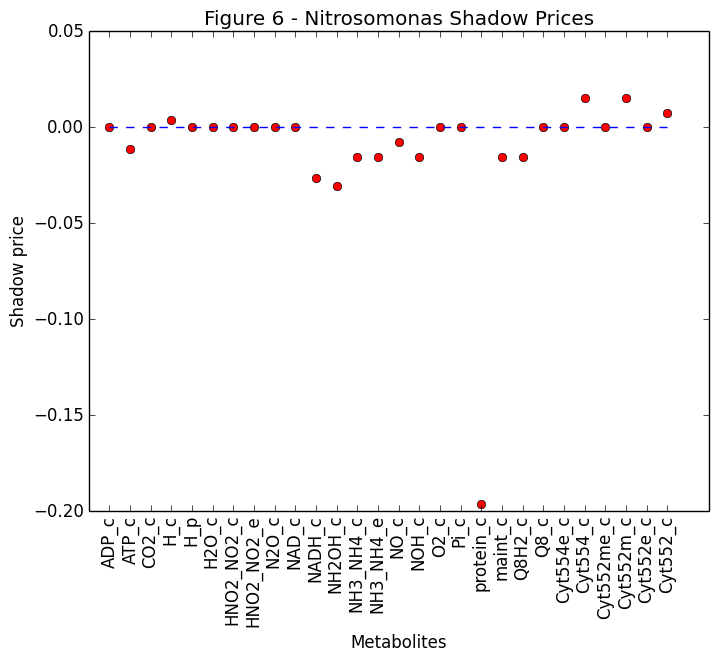Out:
PyObject <matplotlib.text.Text object at 0x319102590>
In :
#plot shadow prices
using PyPlot

dualNB = []
for i in metabolitesNB
push!(dualNB,λNB[i])
end

x = collect(1:length(metabolitesNB))
plot(x, dualNB, "ro", x, zeros(length(metabolitesNB)), "--")
xticks(x, metabolitesNB, rotation="vertical")
xlabel("Metabolites"); ylabel("Shadow price")
title("Figure 7 - Nitrobacter Shadow Prices")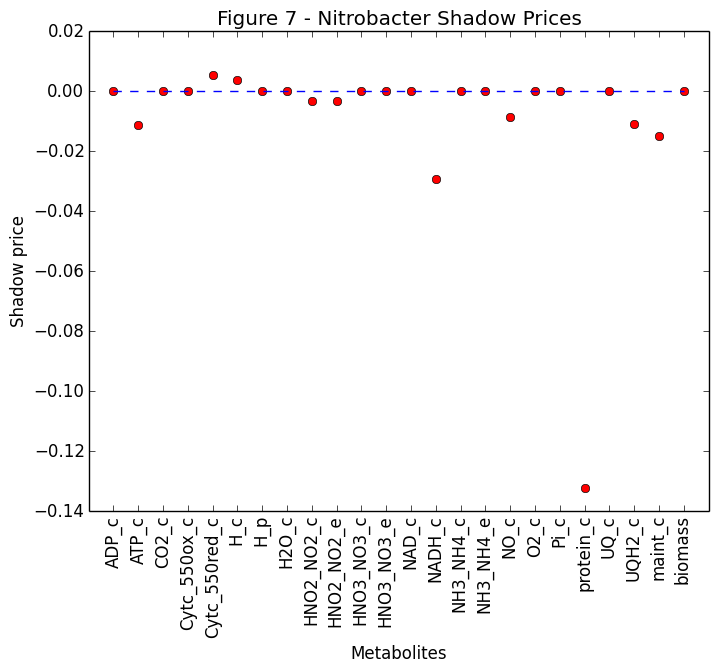Out:
PyObject <matplotlib.text.Text object at 0x319648250>

### 3.B. Phenotypic phase plane summary¶

Figure 8 shows the x-y phenotypic phase plane for Nitrosomonas under varying fluxes for O2 and NH3 uptake. The line of optimality (red) corresponds to where the optimal oxygen and ammonia uptake fluxes provide the maximum biomass yield. From the slope of the isoclines, we can see that two distinct phenotypic phases or "metabolic network states" exist:

• Phase 1: Ammonia is the single limiting substrate (left portion of phase plane, vertical isoclines).
• Phase 2: Oxygen is the single limiting substrate (right portion of phase plane, horizontal isoclines).

The two phenotypic phases can also be observed from the shadow prices shown on Table 2, where both metabolites have a constant value in a given phase. In the ammonia limiting phase, we see that the objective function (biomass growth) is sensitive to changes in NH3, but not O2, whereas the reverse case holds for points in the oxygen limiting phases.

**Table 2**
Metabolite λ(520:100) λ(590:340) λ(200:40) λ(300:200) λ(300:85) λ(350:645) λ(55:530) λ(30:200) λ(185:300) λ(590:590)
NH3 -0.01521 -0.01521 -0.01521 -0.01521 -0.01521 0 0 0 0 0
O2 0 0 0 0 0 -0.01148 -0.01148 -0.01148 -0.01148 -0.01148
Phase 1 1 1 1 1 2 2 2 2 2

Because the metabolic network used in this analysis was very small (30 reactions), no alternate phenotypic phases were found where both substrates became limiting. However, we anticipate that for larger metabolic reconstuctions of Nitrosomonas (e.g. genome-scale model) multiple phenotypic phases would be observed.

In :
#plot PhPP
using PyPlot

function meshgrid{T}(b1::AbstractVector{T}, b2::AbstractVector{T})
m, n = length(b1), length(b2)
b1 = reshape(b1, 1, n)
b2 = reshape(b2, m, 1)
(repmat(b1, m, 1), repmat(b2, 1, n))
end
(X1,Y1) = meshgrid(b1,b2);

#LO line end
a = find(rxn2Flux .<= length(1:range1) )
n = (a[length(a)])

pygui(false)
figure(figsize=(6,5))
contour(growthRates, origin="lower", extent=(0,range1,0,range2), 60, )
plot( b1[1:n], rxn2Flux[1:n], "r-" )
axis("image"); xlabel(rxnID); ylabel(rxnID);
colorbar()
tight_layout();
savefig("PhPP_contour.pdf")
title("Figure 8A - Phenotypic Phase Plane")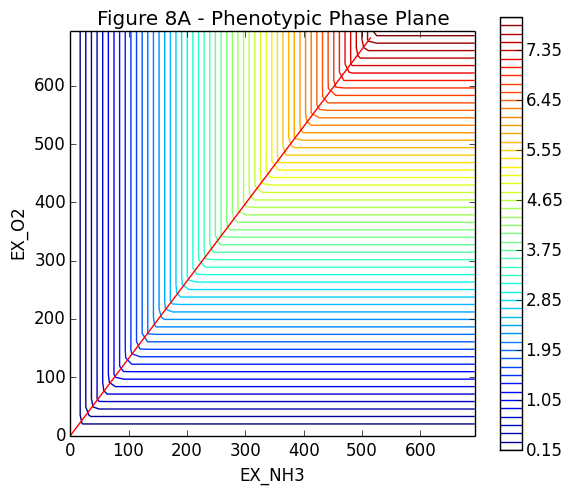Out:
PyObject <matplotlib.text.Text object at 0x319966750>
In :
#plot PhPP
pygui(false)
fig = surf(X1,Y1, growthRates+10, rstride=5, cstride=5,cmap="rainbow")
contour(X1, Y1, growthRates, zdir="z", 50, offset=0, origin="lower" )
#plot( b1[1:n], rxn2Flux[1:n],growthRatesLO[1:n], "r-" )
xlabel("NH3 uptake rate"); ylabel("O2 uptake rate"); zlabel("Growth rate")
tight_layout()
savefig("PhPP.pdf")
title("Figure 8B - 3D Phase Plane")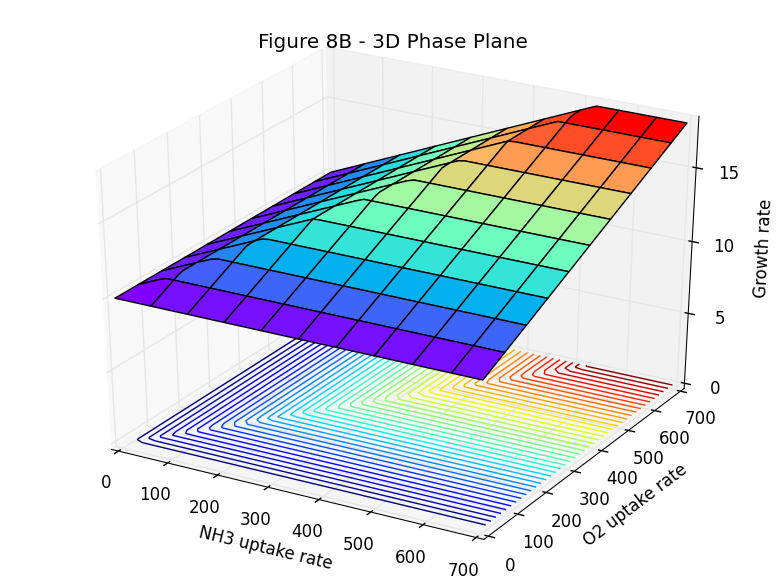Out:
PyObject <matplotlib.text.Text object at 0x319c1b450>

### 3.C. Bioreactor simulation and species competition¶

The ultimate goal of our project was to be able to simulate the population dynamics and nutrient cycling occuring in a nitrifying bioreactor. Figure 9 shows the nutrient profile simulated over one bioreactor batch cycle. As anticipated for nitrification, NH3 is initially consumed followed by a transient accumulation of NO2- and finally NO3- production. Complete nitrification is achieved at a hydraulic retention time of approximately 15 hours, when the biomass concentrations are initially at 1x1010 cells/L.

From the biomass concentrations shown on Figure 10, we can see that N. vulgaris was able to outcome N. winogradskyi for NO2- and proliferate in the system. This is expected becuase both its maximum uptake rate and affinity coefficient for NO2- are better.

A major limitation of the bioreactor model and FBA analyses done here is they do not account for the effects of toxic intermediates. For example, hydroxylamine, nitric oxide, nitrous acid, and ammonia at high concentrations are known to inhibit the growth of nitrifying bacteria (Vadivelu et al., 2006). This could be corrected in the model by adding kinetic terms that explicitly account for toxicity effects.

In :
#plot nutrient concentrations
using PyPlot

x = collect(0:Δt:θ)*24
figure(figsize=(12,4))
plot(x, C[:NH3],"-", x, C[:NO2],"-", x, C[:NO3] )
xlabel("time (hrs)"); ylabel("Concentration (mM)")
legend(["NH3", "NO2", "NO3"], loc="right")
title("Figure 9 - Bioreactor nutrient profile");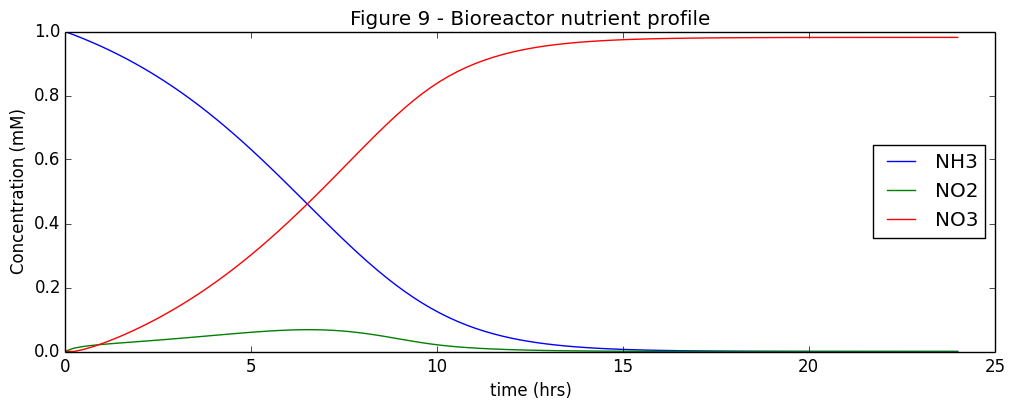In :
#plot biomass growth

x = collect(0:Δt:θ)*24
figure(figsize=(12,4))

plot(x, X[:NS]*1000, "--", x, X[:NB]*1000, "--", x, X[:NV]*1000, "--")
xlabel("time (hrs)"); ylabel("conc. (mg DW/L)")
legend(["NS", "NB", "NV"], loc="lower right");
title("Figure 10 - Biomass growth");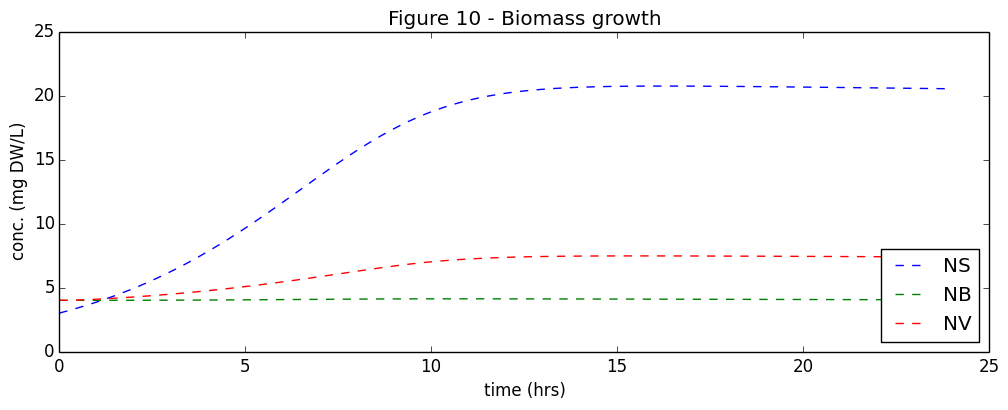## 4. Conclusion¶

The following conclusions can be drawn from this report:

1. The growth phenotype of an organism can be predicted from it's metabolic network using linear programming.
2. The maximum specific growth rates for Nitrosomonas and Nitrobacter agree reasonably well with data from the literature.
3. The metabolite shadow prices from the dual FBA problem can be used to evalute the sensitivity of an organisms maximum growth rate to metabolite imbalances.
4. Metabolite shadow prices can also be used to examine the different phenotypes of an organism via phenotypic phase plane analysis.
5. Dynamics FBA modelling and mathamatical optimization can be used to predict environmental processes, such as nitrification, that rely on mixed-species microbial communities.
6. N. vulgaris outcompetes N. winogradskyi for NO2- under the conditions simulated in this study.

Future work will include the addition of more cooperative species to complete the nitrogen cycle. In particular, the addition of a denitrifying bacteria, such as Thiobacillus dentrificans, Micrococcus denitrificans, or Pseudomonas species would allow for complete conversion of NH3 to nitrogen gas (N2) in our bioreactor system.

Another promising future direction is the application of genome-scale metabolic models. The ~25-30 reaction models used here are a known oversimplication of the actual metabolic network. We predict that incorporating a more complete metabolic reconstruction will reveal alternative phenotypic phases, and allow for a more accurate and comprehensive analysis of microbial metabolic networks.

Finally, we may continue to investigate bi-level dynamic optimzation of the microbial system. Rather than modeling the extracellular enviroment as an ordinary differential equation network, the bioreactor dynamics may also be framed as an optimization problem (see Zomorrodi et al., 2014). This formulation seek to maximize the global biomass concentration subject to constraints generated by "inner" flux balance analysis problems, and represents a nonconvex nonlinear problem.